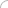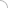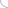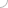# Aeronautical Engineering interview questions and answers

## Aeronautical Engineering interview questions and answers

### Part 1   Part 2   Part 3   Part 4   Part 5   Part 6 What are the characteristics that keep solid and fluid different? What are the objectives of Aerodynamics? What are the sources involved in aerodynamics? What are the conditions given for the two flows to be dynamically similar? What are the differences between continuum flow and free molecule flow? What are the differences between inviscid and viscous flow? ## What are the characteristics that keep solid and fluid different? • When the force is applied tangentially on solid then it experiences a finite deformation and shear stress that is proportional to the deformation. Whereas, when the same shear stress is applied on the surface of fluid then it experiences continuous increasing deformation where, the shear stress is proportional to the rate of change of deformation. • The fluid dynamic is dividend in three different areas. They are as follows: Hydrodynamics (flow of liquids), Gas dynamics (flow of gases) and Aerodynamics (flow of air). Whereas, the state of, solid doesn’t represent any of the stages. ## What are the objectives of Aerodynamics? Aerodynamics deals with the theory of flow of air and it has many practical applications in engineering. There are some objectives that are being used in aerodynamics and these are as follows: • It is used to predict the forces, moments and heat transfer from the bodies that is moving through the liquid. • It deals with the movement of wings or use of the wind force. This way it requires the calculations to be done for the aerodynamic heating of the flight vehicles and the hydrodynamic forces applied on the surface of the vehicle. • It is used to determine the flows that are moving internally through ducts. This way it makes the calculations and measurement of the flow properties that is inside the rocket and jet engines. ## What are the sources involved in aerodynamics? There are two sources that are involved in the case of aerodynamics forces and moments that are on the body. These forces are as follows: • Pressure distribution: this is the distribution that is over the body surface • Shear stress distribution: this is the distribution that is over the body surface These sources are for the body shapes and it doesn’t matter how complex they are. The mechanism that is being used to communicate with the bodies that is moving through a fluid. Both the pressure (p) and shear stress (?) having the dimension force per unit area. This helps the movement of the body through the fluid. ## What are the conditions given for the two flows to be dynamically similar? To measure the dynamicity of the two flows consider two different flow fields over two different bodies. This way the conditions that get generated are as follows: • The streamlined pattern shouldn’t be geometrically similar. • The distribution of the volume over change in volume (V/V8), pressure over change in pressure (p/p8), and time over change in time (T/T8). These changes take place throughout the flow of the field and they remain the same against the common non-dimensional coordinates • The force coefficient remains the same. • There is a similarity in both the flows like the solid boundaries are geometrically similar for both flows. ## What are the differences between continuum flow and free molecule flow? • The flow that is moving over the body i.e. in a circular cylinder of diameter d is the continuum flow, whereas the flow that consists of individual molecules moving in random motion is the free molecule flow. • The mean free path (?) defines the mean distance between the collisions of the molecule and if this path (?) is smaller than the scale of the body measured (d) then the flow of the body is considered as continuum flow. • The path (?) that is of same order as the body scale then the gas molecules then the body surface will have an impact of the molecules and this is known as free molecular flow. ## What are the differences between inviscid and viscous flow? • Viscous flow is the flow in which the molecule moves in random fashion and transfers their mass, momentum and energy from one place to another in fluid. Whereas, an inviscid flow is the flow in which there is no involvement of friction, thermal conduction or diffusion while the molecules are moving. • Inviscid flow consists of the limited influence of friction, thermal conduction and diffusion that is limited to thin region that is limited to the body surface. Whereas, the viscous flows involve the flows that dominates the aerodynamics of the blunt bodies like cylinder. In this the flow expands around front face of cylinder and it separates from the rear surface of it.  Part 1   Part 2   Part 3   Part 4   Part 5   Part 6 (adsbygoogle = window.adsbygoogle || []).push({}); Write your comment - Share Knowledge and Experience Discussion Board Thermodynamic Question Durgesh 08-23-2016 01:43 PM   (adsbygoogle = window.adsbygoogle || []).push({}); (adsbygoogle = window.adsbygoogle || []).push({});Interview questionsLatest MCQs » General awareness - Banking » ASP.NET » PL/SQL » Mechanical Engineering » IAS Prelims GS » Java » Programming Language » Electrical Engineering » English » C++ » Software Engineering » Electronic Engineering » Quantitative Aptitude » Oracle » English » Finance Home | About us | Sitemap | Contact us | We are hiring © Copyright 2016. All Rights Reserved. Terms of use  |  Follow us on Facebook!